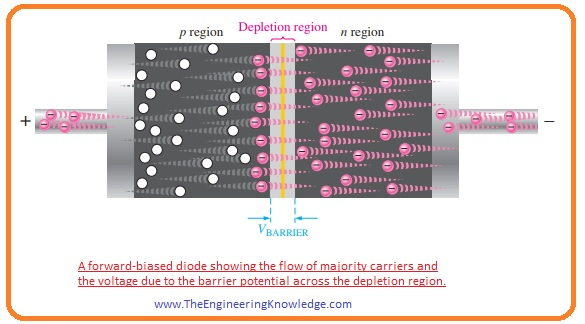Hello, friends, I hope you all are doing great. In today’s tutorial, we will discuss the Difference Between Diode and Thyristor. The main difference between diode and thyristor is that diode has 2 terminals and used as a rectifier for converting AC to DC and as a switch. While thyristor has 2 terminals and operates as a switch.

Both diode and thyristor are semiconductor devices and constructed with the combination of p and n types of materials. In today’s post, we will have a detailed look at both diode and thyristor and find their differences. So let’s get started with the difference between diode and thyristor.

#### Difference Between Diode and Thyristor

Diode

• It is a semiconductor device that used for rectification or ac to dc conversion.
• There are different types of diode like Zener, step recovery.
• there are two types of mode of operation in diode first one is forward biased and the second one is reverse biased
• Its operating voltage value for the case of silicon is 0.7 volts and germanium is 0.3 volts.
• It uses as a rectifier, in clamping circuits, etc
• In reverse biased anode is connected to the negative terminal and cathode to the positive terminal of the battery.
• The current flow in the diode due to two types of charge carries the first one is a hole and the second one is electrons.
• There are two parts of the diode first is positive and the second is negative has electron as carriers and P has holes
• The material used for the construction of a diode is silicon and germanium.
• Its reverse breakdown voltage value is less large than the LED.

Thyristor

• This controlled electronic turn on a module that needs a trigger pulse for operation.
• It has four layers NPNP or PNPN.
• It is analogs to the SCR in some cases
• It operates as a bistable switch in the case when the gate has a current connection and operation continues in case of voltage about the component is not in the reverse-biased state.
• It used to control the high value of current through 2 points getting involved with the control point
• It exists in the two categories of design armaments.
• In firestone is two terminal and the second one is three terminals.
• They have applicaoin where high value of volts and current are involved.
• Used to control ac current and control the zero-cross operation.
• Its operarion is synchronous in case when gets on it operates in phase to the voltage given at its terminals.
• There are three conducting modes it has reverse, forward and forwards conduction mode
• It has three junctions.
• It has three terminals anode-cathode and gate.
• It used in phase fires controller ciuts
• Its power handling ability is better than the diode.
• It required a large value of voltage than the diode for operation.
• It is expensive than the diode.
• It is a heavyweight device.

#### Diode Forward Biasing

• Biasing is a process in which input supply is provided at the terminals of diode.
• In forwarding biased condition current flows due to movement of electrons and holes across the PN junction.
• In given figure, you can see that diode is attached with the dc voltage source in the forward-biased direction.• The voltage biased provided by the external dc source is represented as Vbias.
• You can see that in the circuit resistance is connected in series with battery and diode it is for limit the value of current and known as current limiting resistance.
• There are 2 conditions should be followed for the forward biased condition.
• The first is to connect the anode of a diode with the positive terminal of the battery and cathode with the negative terminal of the battery.
• In the second condition, the value of the voltage source connected with the diode should be larger than the potential barrier.
• The behavior of diode after forward biasing is shown in a given figure.• As we know that similar charges oppose each other so negative terminal apply force on the electrons in N region and they start to move towards the PN junction.
•  Due to the movement of electrons current flows through the electrons.
• As the negative terminal of the battery continuously providing energy to the electrons so they have enough energy to cross the potential barrier and enters in the P region and combine with the holes after losing their energy.
• As opposite charges attract each other so positive terminal of battery also attracts the electrons in P region an electron starts to move towards that terminal.
• As electron moved from N-region to  P Region so holes also created in the N region that moves towards the negative terminal of the battery.
• In this process, we can conclude that current in forwarding biasing condition flows due to the flow of electrons and holes.

That is all about difference between diode and thyristor. I tried my level best to make simple this post for you if you have any query ask in comments. Thanks for reading. have a good day.